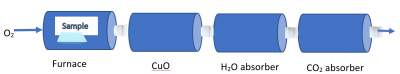# Combustion Analysis

## Introduction

Combustion is burning a material in air (i.e. oxygen). This process is mainly used for compounds containing C & H as their elements.When combusted in air and with the help of a catalyst (to enhance the process), it produces CO2 and H2O.

To calculate the amount of H and C in the original sample, the amount of H2O and CO2O produced are determined after being absorbed by H2O and CO2 absorbers.

### Example

When 0.255 g of Isopropyl alcohol (has C,H,O) is combusted In Air, it produces 0.561 g CO2 and 0.306 g H2O

Question: What is the empirical formula of the alcohol?

Solution:
• In a similar question, the exact molecular formula may be asked about if the molecular weight is given:
• Hence, we use the empirical formula and its weight as well as the molecular weight to get the common number.
• Then, multiply the empirical formula by the common number to get the molecular formula, according to the following relation:
• Molecular Formula / Empirical Formula = Molecular Formula Weight / Empirical Formula Weight = Common Number